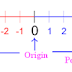## What is an Integer in Maths?

An integer is a number which has no decimal or fractional part.  It has set of negative and positive numbers, including zero.

### Integer Examples:

-10, 0, 11, 25, -8, 90, and 4587.

Integer Types:
Positive Integers: A number is greater than zero defined as positive Integers.
Positive Integer Example: 1, 2, 3 . . .

Negative Integers: A number is  less than zero defined as negative Integers.
Negative integers Example: -1, -2, -3 . . .

Zero is defined as neither negative nor positive integer. It is a whole number.

### negative and positive number line

A number line which we represents integers on a straight line in a graphical manner.

### Integer Operations

We can perform arithmetic operations on the integers. Most basic operations are

2. Subtraction Integers

3. multiplying integers

4. divide integers

1. Adding integers:  Adding integers,  we can find the sum of two or more integers. The result of sum value will be increased or decreased depending on the integer sign value i.e. , positive or negative value.

Example 1:  Find the sum of 3+6

Solution: 3 and 6 both positive integers and result will be 9.

Example 2:  Find the sum of 3+(-10)

Solution: An expression has two different sign to the numbers. First number, 3 is positive number and second, -10 is a negative number. When we do the sum, result will be -7.

Example3:  Find the sum of (-6)+(-20)
Solution: An expression that has same sign of numbers i.e., negative number, perform addition on numbers, result value also we get as negative number that is -26.

2. Subtraction Integers: Subtraction integers,  we can find the difference between two or more integers. The result of difference value will be increased or decreased depending on the integer sign value i.e. , positive or negative value.

Example :  Find the value of 5 - 8.
Solution: An expression that contain two numbers, first number is smallest number than the second number.  In this case we get result value has negative sign.  That is -3. Generally 8-5 gives 3, because of First number larger than the second number and differ value is 3.

3 Multiplying integers:
Multiply is nothing but a repetition process. We perform multiply on the number and specify the  value how many times repeat it. For example 5x3 is a mathematical expression, it gives 15 as result. In that case 5 is a number and 3 is repeatation value. That means we are counting the number 5 in three times i.e 5+5+5 (5 is repeating 3 times) that will give 15.

The table shows product of signs and it result of sign value.

 Product of Signs Result Example + × + + 5 × 3 = 15 + × - - 5 × (-3) = -15 - × + - (-5) × 3 = -15 - × - + -5 × -3 = 15

4. Division Integers

Division of integers means find out one number is determine how many times a number is contained into another number.  This is opposite to multiplication process. Example, 36 ÷ 6 that gives result is 6.   Here, we are finding out how many times 6 is contained in 36. (i.e, 6+6+6+6+6+6 , 6 is repeated 6 times that gives 36.)

There are few cases, we should know that is when we perform division operation on the sign of numbers result should we get as either positive or negative sign.
case 1: positive ÷ positive = positive
case 2: negative ÷ negative = positive
case 3: negative ÷ positive = negative
case 4: positive ÷ negative = negative

What is an Integer in Maths?Reviewed by SSC NOTES on January 28, 2022 Rating: 5